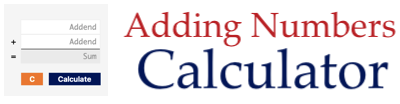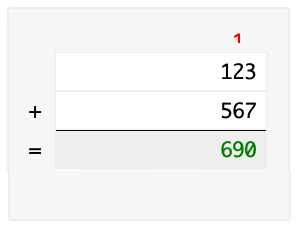This useful Adding Numbers Calculator can add up any two numbers and show you how to do it with long addition and carryovers.

Please enter your two addends so we can calculate the sum for you.

Below is an example of how the Adding Numbers Calculator works and how to add numbers using the long addition method. For our example, we will use 123 plus 567.

When you type in 123 for the first number (first addend) and 567 for the second number (second addend) and press calculate, this will be your result:Here are step-by-step instructions showing you how our Adding Numbers Calculator used long division to get the answer.

1) 3 + 7 = 10. Enter 0 in green at the bottom and 1 in red carryover.

2) 2 + 6 + 1 = 9. Enter 9 in green at the bottom.

3) 1 + 5 = 6. Enter 6 in green at the bottom

The answer is in green at the bottom. There you have it folks. Enjoy!